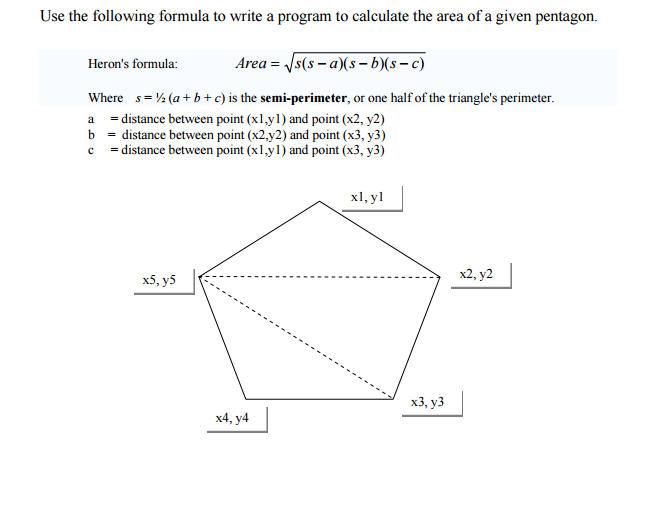Visual Basic, Calculate area of a Pentagon1) Make sure the entered values are positive integers
2) Make sure you have five different points
3) Please use ActiveForm to know the width and height of the form where the pentagon is drawn. The five points should be less than width and height of the form.
4) Any three points should not be on the same line.
Basically, we are checking that the slopes between point 1 and point 2 and point 1 and point 3 match. Slope is change in y divided by change in x, so we have:
y1 - y2 y1 - y3
------- = --------
x1 - x2 x1 - x3
Cross multiplying gives (y1 - y2) * (x1 - x3) == (y1 - y3) * (x1 - x2);